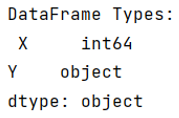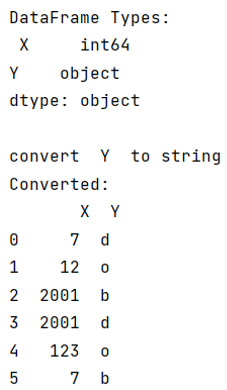# Strings in a DataFrame, but dtype is object

The solution for "Strings in a DataFrame, but dtype is object".
Submitted by Pranit Sharma, on June 28, 2022

Pandas is a special tool that allows us to perform complex manipulations of data effectively and efficiently. Inside pandas, we mainly deal with a dataset in the form of DataFrame. DataFrames are 2-dimensional data structures in pandas. DataFrames consist of rows, columns, and the data. The Data inside the DataFrame can be of any type.

Pandas is quite easy to understand but sometimes when we insert a string inside a DataFrame, and dtpes says that the string is an object, we might have taught why it is not written 'string'.

The object data type is a part of the NumPy array, it represents an array that must have the same size in bytes.

This can also be manipulated, let us understand with the help of an example,

To work with pandas, we need to import pandas package first, below is the syntax:

```import pandas as pd
```

## Python code for 'strings in a DataFrame, but dtype is object'

```# Importing pandas package
import pandas as pd

# Creating dictionary
d = {
'X':[7,12,2001,2001,123,7],
'Y':['d','o','b','d','o','b']
}

# Creating dataframe
df = pd.DataFrame(d)

# Display DataFrame types
print("DataFrame Types:\n",df.dtypes,"\n")
```

Output:```# Importing pandas package
import pandas as pd

# Creating dictionary
d = {
'X':[7,12,2001,2001,123,7],
'Y':['d','o','b','d','o','b']
}

# Creating dataframe
df = pd.DataFrame(d)

# Display DataFrame types
print("DataFrame Types:\n",df.dtypes,"\n")

# Manipulate the column
for c in df.columns:
if df[c].dtype == object:
print("convert ", df[c].name, " to string")
df[c] = df[c].astype(str)

# Display modified DataFrame
print("Converted:\n",df)
```

Output: